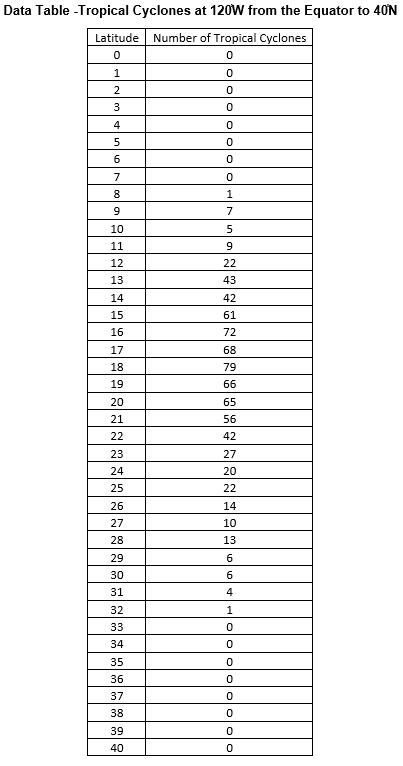## Tropical Cyclone Counts Create Box Plot

The purpose of this lesson is for students to create, a box plot and evaluate the spread of the data. This is part of the Tropical Cyclone Counts Graphing Bundle and can be completed independently or with the other activities in the bundle.

### Mini Lesson

Review box plots briefly.

1. A box plot is a type of graph which shows variability within a group. It shows the maximum, minimum, median, upper and lower quartile of the data.
2. It also shows the spread of the data. Each section of the plot shows 25% of the data.
3. Box plots are very useful for comparing data sets to each other.
4. Box plot are most often displayed horizontally. Box plots created in software programs are sometimes vertical. Either representation is valid.
5. The types of questions that might be answered with a box plot include:
• What is the range of the populations of different countries?
• What is the median test score in a class?
• What is the range for the top 25% of the scores on a test? The bottom 25%?
• What is the most/least chocolate chips the bags sampled?
• How large is the spread of the data?

Plan your Box plot

1. Use the data table provided to calculate the following. Remember to order the data from least to greatest before doing the calculations.

• Median

• Minimum

• Maximum

• First Quartile

• Third Quartile

• Inter-Quartile Range

2. What label will you use for the scale?

3. How many squares of the graph paper will you use for each unit?

4. How many squares will you need for the scale?

5. What title will you use?

6. Will you draw it vertically or horizontally?

Create the Box plot Using the Answers to the Planning Questions

1. Draw the scale using the graph paper provided.

2. Label the scale.

3. Add the title.

4. Using the minimum, maximum, median, lower and upper quartiles, draw the box plot.

5. The plot will be analyzed in Mini Lesson 3 Question Set 1.